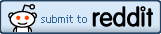## Friday, 20 September 2013

### Playing With EvolutionProbability = 1:100,000. Advantage factor = 51%
One of the things people sometimes find difficult to understand about evolution is the idea of a species evolving up an improbability gradient so that something which was highly improbable becomes the norm over time because of the multiplying effect of natural selection. Creationist frauds will point at an evolved characteristic and come up with some spurious notional estimate of the probability of it evolving 'by random chance alone' and dismiss the idea as too preposterous for words. It's the equivalent of standing at the bottom of a cliff and declaring that life can't possibly exist at the top of it because nothing could jump that high in a single bound.

Indeed, this is one of the stocks in trade of professional Discovery Institute liars who earn a living misrepresenting the mathematics of biology as part of the Wedge Strategy to discredit science because of the way an understanding of the Universe undermines religious superstition and makes it harder to retain the unelected political power that controlling the people's religion gives them.

Richard Dawkins devoted an entire book, Climbing Mount Improbable to explaining the idea of the improbability gradient.

Here's a very crude, simple spreadsheet for modelling in very simple terms how this works. You'll need Microsoft Excel© and the ability to create a simple chart with it.

Here's what you do:

Open up a new Excel spreadsheet and type the following into the given cells. Have particular care with the formulae.

CellContent
A1Population
A2Advantage Factor
A3Initial number of mutants
B1100000
B251%
B31
D1Generation
E1Population A
F1Population a
G1Percentage of A
H1Percentage of a
D2=Row()-2
E2=B1-F2
F2=B3
G2=100*E2/(E2+F2)
H2=100*F2/(E2+F2)
D3=Row()-3
E3=(\$E\$2+\$F\$2)*(E2*(100%-\$B\$2))/((E2*(100%-\$B\$2))+(F2*\$B\$2))
F3=(\$E\$2+\$F\$2)*(F2*\$B\$2)/((F2*\$B\$2)+(E2*(100%-\$B\$2)))
G3=100*E3/(E3+F3)
H3=100*F3/(E3+F3)
Now copy down all the formulae in columns D through F to at least row 500 or as many more rows as you wish. You can always add more later.

What this has done is set up a model for calculating the average number of alleles in each generation in a population of 100000 if the mutation (a) gives a differential probability of reproducing of 51:49 compared to the normal form (A). It also gives you the same figures expressed as a percentage of the population. It replicates what would happen if the probability of the initial mutation was one in one hundred thousand but ignores any future mutations. You can play with these settings to see what happens in a larger population or with a different probability. For example, to test out a 1 in a million chance, change the value in B1 to 1000000 and leave B3 with a value of 1. To change the modelled advantage change the parameter in B2. You may need to adjust the number of rows.

To appreciate fully how the numbers change over time, create a chart and plot either columns E and F, or Columns G and H, with column D as the X-axis. You can then make changes and see how the shape of the graph changes. I find a stacked bar-chart gives the best result but you can experiment with others.

If you want to use this as a starting model and build in things like random drift where there are no significant selection pressures, population growth from a small founder population or several co-evolving genes interacting, be my guest. Your skill and patience with Excel will be greater than mine, but I would be interested in seeing the results.

Enjoy.#### Post a Comment

Obscene, threatening or obnoxious messages, preaching, abuse and spam will be removed, as will anything by known Internet trolls and stalkers, by known sock-puppet accounts and anything not connected with the post,

A claim made without evidence can be dismissed without evidence. Remember: your opinion is not an established fact unless corroborated.

Sady, the spammer is back so you'll need to sign on to post comments.